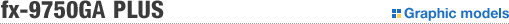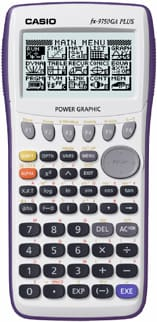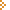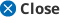Close upNew Design & High Contrast Display !!! All the powerful features and functions of the CASIO Graphic Scientific Calculator plus program function, advanced statistics, financial function, and more! *fx-9750GA PLUS has equipped with the same function as fx-9750G PLUS.Graphic Drawing of rectangular coordinate, polar coordinate, parametric, inequality and other types of graphs, as well as support for visual analysis of functions.Graph Solve Functions can be graphed to obtain roots, maximums, minimums, y-intercepts, points of intersection, coordinates for any point, integral value approximations, and more.Sketch Lines, normal lines, points, line segments, and comment text can be incorporated directly into graphs.Dual Graph The screen can be split in two for simultaneous display of two graphs for comparison of two different graphs, to view an enlarged version of the same graph, and for other purposes that help in analysis of graphs.Graph to Table The screen can show a graph on one side and a numeric table on the other. An easy operation inputs the coordinate values at the flashing point on the graph into the numeric table.Dynamic Graph Dynamic Graph causes the shape of the graph to continually change in accordance with changes in function parameter values within a specific range.Table & Graph Numeric tables can be created for graphing scientific functions. The ability to draw graphs using discrete data calculated from the function makes it possible to easily grasp the properties of the function.Recursion Any two of the three recursion formulas listed below can be input to generate a numeric table for graphing.• General term of expression {an}, which is formed by an, n • Linear binomial recursion, which is formed by an + 1, an, n • Linear trinomial recursion, which is form by an + 2, an + 1, an, nConics The following four types of implicit function graphs can be drawn using built-in functions.• Parabolic graph • Circle graph • Elliptical graph • Hyperbolic graph[ 900 Functions ] [ 28,000 bytes RAM ] [ 21 Characters by 8 Lines ] [ 10 Digits or 10+2 Digits ] [ Icon Menu ] [ Dot Matrix ]• Large display (128 X 64 dots) • Graphic functions (Rectangular coordinate graph, Polar coordinate graph, Parameter graph, Inequality graph, Integration graph, Statistical graph) • Dynamic graph • Dual graph • Conic section graph • Regression graph (Logistic regression graph, Sinusoidal regression graph, Linear regression graph, and more) • Graph solve • Integrations • Differential and quadratic differential calculations • Complex number calculations • Table and graph • Recursion graph • List-based statistics • Advanced statistics • BASIC-like program functions • Linear equations from two to six unknowns • Quadratic equations, Cubic equations • Matrix operations • Base conversions/ calculations • Financial function • Data communications • Power supply: Main - Four AAA size batteries (R03/LR03) Backup - One lithium battery (CR2032) • Approximate battery life: Main - 240 hours with R03/ 420 hours with LR03 (continuous display of main menu) backup-2 years • Dimensions: 21.6H X 87.0W X 179.5D mm • Approximate weight: 205 g